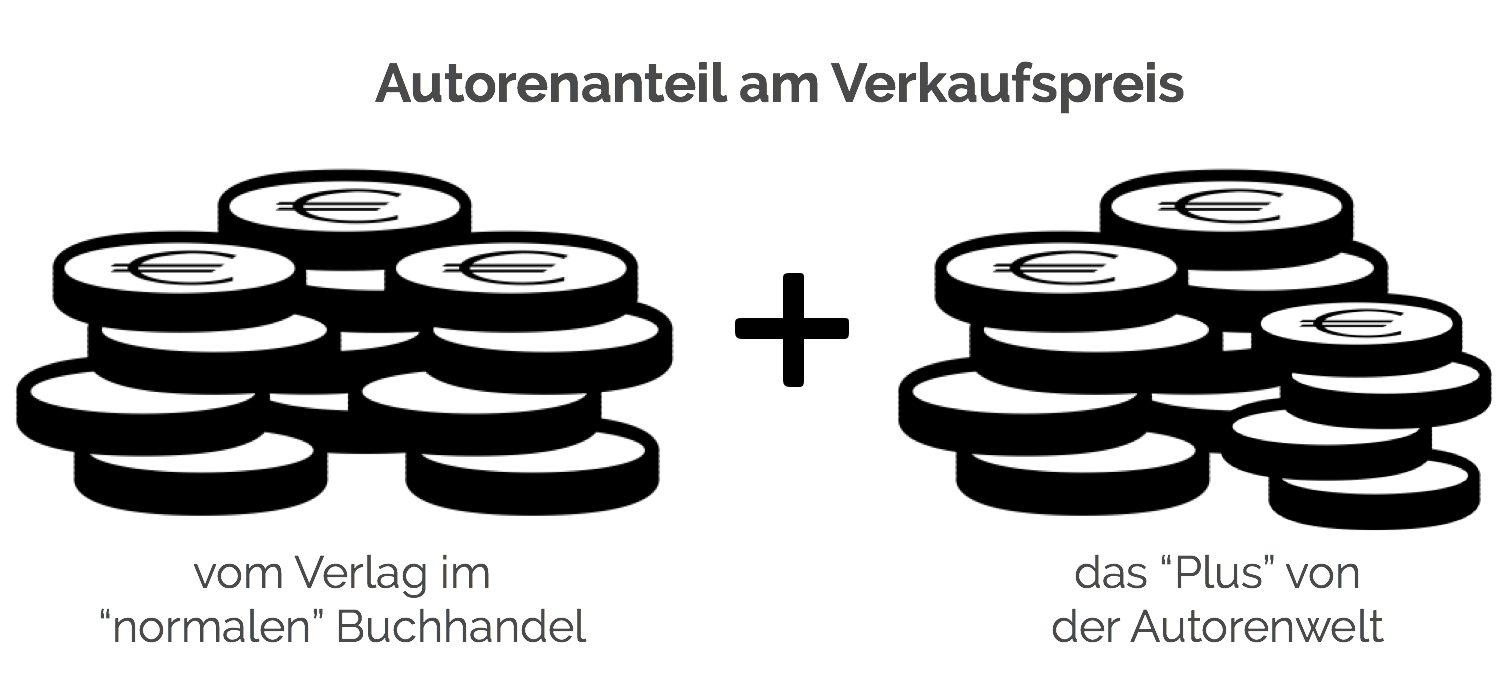# Vector Field Reconstruction: Vector Field, Differential Equation, Mathematical Model, Dependent and Independent Variables, Ordinary Differential Equation, Voltage, Parameter, Resistor, Chaos

Taschenbuch - 9786130354466
45,00 €
• Versandkostenfrei
• Hinweis: Print on Demand. Lieferbar innerhalb von 7 bis 10 Tagen
• inkl. MwSt. & Versandkosten (innerhalb Deutschlands)
• Hinweis: Print on Demand. Lieferbar innerhalb von 7 bis 10 Tagen
• inkl. MwSt. & Versandkosten (innerhalb Deutschlands)

## Autorenfreundlich Bücher kaufen?!## Beschreibung

High Quality Content by WIKIPEDIA articles! Vector field reconstruction is a method of creating a vector field from experimental or computer generated data, usually with the goal of finding a differential equation model of the system. A differential equation model is one that describes the value of dependent variables as they evolve in time or space by giving equations involving those variables and their derivatives with respect to some independent variables, usually time and/or space. An ordinary differential equation is one in which the system's dependent variables are functions of only one independent variable. Many physical, chemical, biological and electrical systems are well described by ordinary differential equations. Frequently we assume a system is governed by differential equations, but we do not have exact knowledge of the influence of various factors on the state of the system. For instance, we may have an electrical circuit that in theory is described by a system of ordinary differential equations, but due to the tolerance of resistors, variations of the supply voltage or interference from outside influences we do not know the exact parameters of the system.

## Details

 Verlag Betascript Publishers Ersterscheinung Februar 2010 Maße 220 mm x 150 mm x 9 mm Gewicht 237 Gramm Format Taschenbuch ISBN-13 9786130354466 Auflage Nicht bekannt Seiten 148

## Schlagwörter

• american express
• apple pay
• bitcoin
• bitcoin cash
• ethereum